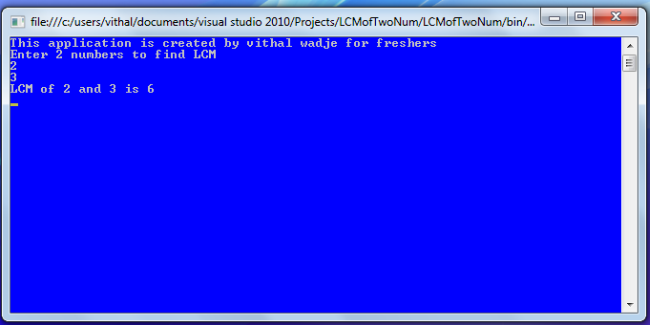Featured

# Find LCM of Two Numbers Using C#

In this article I will explain how to determine the Lowest Common Multiples of two numbers in a  C# console application. This type of question might be asked by an interviewer in a .Net position related interview for beginners.
So considering the preceding requirement and numerous emails sent to me from beginners I have decided to write this article with the basics and specially focusing on the beginner, student and whoever might face this type of question in an interview.
What is a LCM ?
LCM Stands for Lowest Common Multiple of two numbers.
e.g
First Number : 2 -- 2,4,6,8,10,12,14,16,18,20...
Second No : 3 -- 3,6,9,12,15,18,21,24,27,30...

Then the LCM of 2 and 3 is: 6.
In the example above you clearly see that two numbers (2 and 3) are used and I have multiplied them with their same range number that is 2,4,6..or 3,6,9.. etc. Then in the example above the Lowest Common Multiples between the numbers 2 and 3 are 6.
Now I hope that we completely understand what is meant by LCM, next I will explain how to do it step-by-step, as in the following.
Open Visual Studio from Start - - All programs -- Microsoft Visual Studio.
Then go to to "File" -> "New" -> "Project..." then select "Visual C#" -> "Windows" -> "Console application".

After that specify the name such as LCM two_numbers or whatever name you wish and the location of the project and click on the OK button. The new project is created.

And use the following code in the program.cs class file:

```using System;
namespace LCMofTwoNum
{
class Program
{
public static int findLCM(int a, int b) //method for finding LCM with parameters a and b
{
int num1, num2;                         //taking input from user by using num1 and num2 variables
if (a > b)
{
num1 = a; num2 = b;
}
else
{
num1 = b; num2 = a;
}

for (int i = 1; i <= num2; i++)
{
if ((num1 * i) % num2 == 0)
{
return i * num1;
}
}
return num2;
}

static void Main(string[] args)
{
int num1, num2;
Console.WriteLine("This application is created by vithal wadje for beginners");
Console.WriteLine("Enter 2 numbers to find LCM");
int LCMresult = findLCM(num1, num2); //passing user input value to method using num1 and num2 variable

Console.WriteLine("LCM of {0} and {1} is {2}", num1, num2, LCMresult);

}
}
}
```
In the program above I have clearly explained in comments which statement is used for which purpose. Now run the program with the two integers 2 and 3 as input; then the output will be as follows:Summary